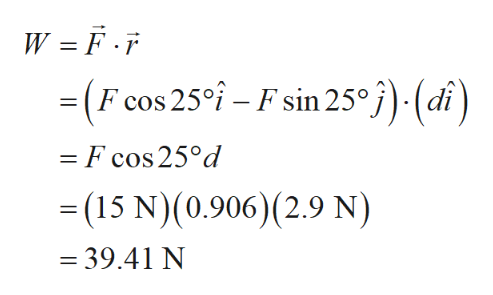# A block of mass m = 2.50 kg is pushed d = 2.90 m along a frictionless horizontal table by a constant applied force of magnitude F = 15.0 N directed at an angle θ = 25.0° below the horizontal as shown in the figure below.A block labeled m is on a horizontal surface. An arrow labeled vector F points downward and to the right at an angle θ above the horizontal, and acts upon the upper left corner of the block. A faded image of the block is a distance d to the right of the block. (a) Determine the work done by the applied force. J(b) Determine the work done by the normal force exerted by the table. J(c) Determine the work done by the force of gravity. J(d) Determine the work done by the net force on the block. J

Question
15 views

A block of mass

m = 2.50 kg

is pushed

d = 2.90 m

along a frictionless horizontal table by a constant applied force of magnitude

F = 15.0 N

directed at an angle

θ = 25.0°

below the horizontal as shown in the figure below.

A block labeled m is on a horizontal surface. An arrow labeled vector F points downward and to the right at an angle θ above the horizontal, and acts upon the upper left corner of the block. A faded image of the block is a distance d to the right of the block.

(a) Determine the work done by the applied force.
J

(b) Determine the work done by the normal force exerted by the table.
J

(c) Determine the work done by the force of gravity.
J

(d) Determine the work done by the net force on the block.
J

check_circle

Step 1

Hello. Since your question has multiple sub-parts, we will solve first three sub-parts for you. If you want remaining sub-parts to be solved, then please resubmit the whole question and specify those sub-parts you want us to solve.

Given information:

Step 2

Here, d is the horizontal distance moved by block.

Consider the FBD below.

Step 3

(a) Write the formula for work(W) and plug the required values to de...help_outlineImage TranscriptioncloseW = F.F = (F cos 25 - F sin 25°j)-(di) - F cos 25°d -(15 N)(0.906) (2.9 N) = 39.41 N fullscreen

### Want to see the full answer?

See Solution

#### Want to see this answer and more?

Solutions are written by subject experts who are available 24/7. Questions are typically answered within 1 hour.*

See Solution
*Response times may vary by subject and question.
Tagged in

### Science